# Axis Layout and Appearance

This topic describes how to modify an axis‘s layout and change its color and thickness. See the Tickmarks, Grid Lines, and Interlacing document for information on how to customize axis tickmarks’ and grid lines’ appearance.

## How to Reverse an Axis Labels’ Order

The Chart Control labels an x-axis from left to right, and a y-axis from bottom to top. You can reverse an x-axis to label it from right to left, and a y-axis from top to bottom. The following images show a y-axis’s labels in the default and reversed order:

Default labels’ order Reversed labels’ order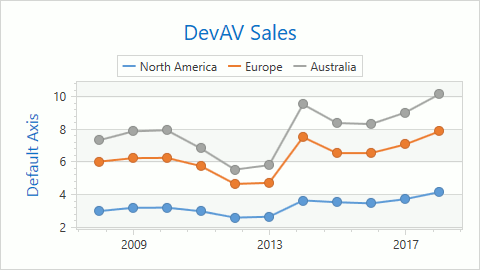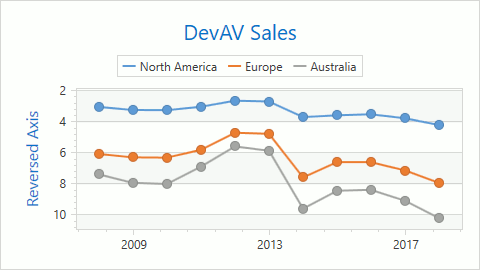Use the following example to display a y-axis’s labels in reverse order:

### Markup

``````<dxc:XYDiagram2D.AxisY>
<dxc:XYDiagram2D.AxisY>
<dxc:AxisY2D Reverse="True"/>
</dxc:XYDiagram2D.AxisY>
<!-- Other diagram settings. -->
</dxc:XYDiagram2D>
``````

### Code

``````<dxc:XYDiagram2D x:Name="diagram">
<!--...-->
</dxc:XYDiagram2D>
``````
``````using DevExpress.Xpf.Charts;

public MainWindow() {
//...
diagram.AxisY = new AxisY2D { Reverse = true };
}
``````

## How to Change an Axis Position

You can display an x-axis on the bottom or top of a chart’s plot area, and a y-axis on the left or right. To change an axis’s position, use the Axis2D.Alignment property. The following images demonstrate different axis alignments:

Value The Example Image
Center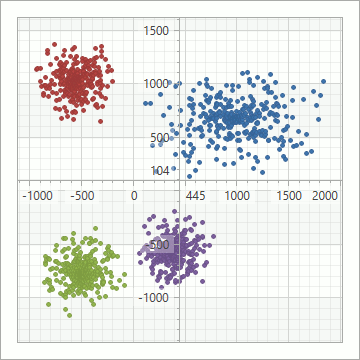Far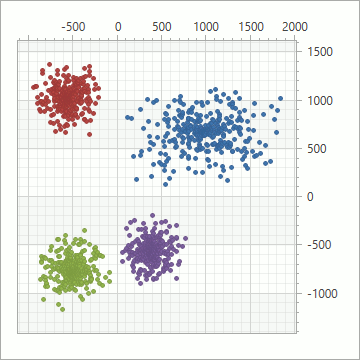Near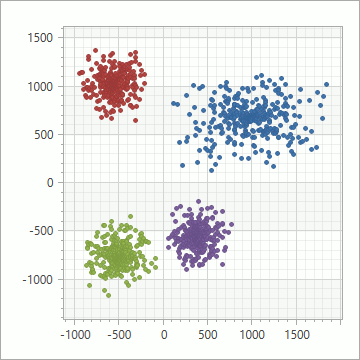Zero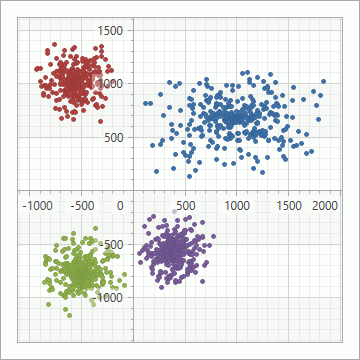The example below shows how to set the Far alignment for a y-axis:

### Markup

``````<dxc:XYDiagram2D>
<dxc:XYDiagram2D.AxisY>
<dxc:AxisY2D Alignment="Far"/>
</dxc:XYDiagram2D.AxisY>
<!-- Other diagram settings. -->
</dxc:XYDiagram2D>
``````

### Code

``````<dxc:XYDiagram2D x:Name="diagram">
<!--...-->
</dxc:XYDiagram2D>
``````
``````using DevExpress.Xpf.Charts;

public MainWindow() {
//...
diagram.AxisY = new AxisY2D { Alignment = AxisAlignment.Far };
}
``````

## How to Customize Axis Appearance

You can modify an axis’s thickness and the brush that is used to draw the axis.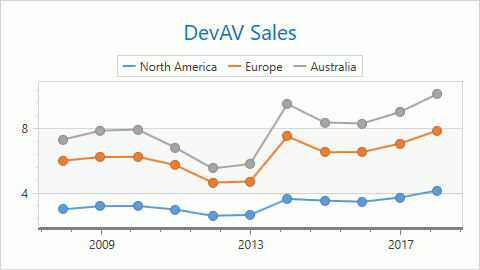The markup below shows how to modify the x-axis’s appearance as shown in the previous image:

### Markup

``````<dxc:XYDiagram2D>
<dxc:XYDiagram2D.AxisX>
<dxc:AxisX2D Brush="Gray"
Thickness="3"/>
</dxc:XYDiagram2D.AxisX>
<!-- Other diagram settings. -->
</dxc:XYDiagram2D>
``````

### Code

``````<dxc:XYDiagram2D x:Name="diagram">
<!--...-->
</dxc:XYDiagram2D>
``````
``````using DevExpress.Xpf.Charts;
using System.Windows.Media;

public MainWindow() {
//...
diagram.AxisX = new AxisX2D { Brush = Brushes.Gray, Thickness = 3 };
}
``````

You can also use DevExpress themes to modify chart elements’ appearance. Refer to Themes for information on how to apply a theme to an application.